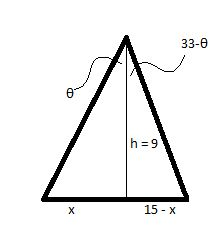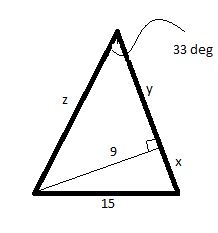# Triangle \bigtriangleup ABC is given where \angle A=33^\circ, \text{ and } a = 15 in, and...

## Question:

Triangle {eq}\bigtriangleup ABC {/eq} is given where {eq}\angle A=33^\circ, \text{ and } a = 15 {/eq} in, and the height, {eq}h = 9 {/eq} in. How many distinct triangles can be made with the given measurements? Explain.

## Solutions of Triangle:

This problem involves the solving of a triangle with given parameters. We need to identify how many distinct triangles can be drawn with the given parameters. The idea is to use trigonometric and inverse trigonometric functions and identities to give a solution of the required triangle. Note, every possible triangle must be drawn as a possibility before starting to solve the problem.

Given, Triangle {eq}\displaystyle \bigtriangleup ABC {/eq} is given where {eq}\displaystyle \angle A=33^\circ, \text{ and } a = 15 {/eq} in, and the height, {eq}\displaystyle h = 9 {/eq} in.

(A) So, we can draw a figure as -Thus, we can say that

{eq}\displaystyle \theta = \tan^{-1} \frac{x}{9} {/eq}

and,

{eq}\displaystyle 33 - \theta = \tan^{-1} \frac{15-x}{9} {/eq}

Thus, upon adding the two equations we have -

{eq}\displaystyle 33 = \tan^{-1} \frac{x}{9} + \tan^{-1} \frac{15-x}{9} {/eq}

So, we can also say that

{eq}\displaystyle 33 = \tan^{-1} \frac{ (x/9) + (15-x)/9 }{1 - (x/9)(15-x)/9} = \tan^{-1} \Big( \frac{ 135}{x^2 -15x + 81} \Big) {/eq}

But since, x and (15-x) are actually distances, they can not be negative. Hence,

{eq}\displaystyle x \in [ 0,15] {/eq}

But the minimum value of RHS that it {eq}\displaystyle \tan^{-1} \Big( \frac{135}{x^2 -15x +81} \Big) {/eq} occurs are x = 0 or x = 15, and is equal to {eq}\displaystyle 59.036 ^\circ {/eq}

Thus, LHS and RHS can never be equal. Hence, no such triangle exists.

(B) We can also draw the figure as -We can find the value of x as -

{eq}\displaystyle x^2 = 15^2 - 9^2 \Rightarrow x = 12 {/eq} in

Also, we can find the value of y and z as

{eq}\displaystyle y = 9 \cot 33^\circ = 13.86 \\ \displaystyle z = 9 \csc 33^ \circ = 16.52 {/eq}

So, the triangle with the dimensions (15, 16.52, 25.86) can be a solution of the triangle.

Note, no other possible triangles can be drawn.